# [Neural Networks and Deep Learning] week4. Deep Neural Network

[TOC]

## Deep L-layer neural network

Layer counting:

• input layer is not counted as a layer, "layer 0"
• last layer (layer L, output layer) is counted.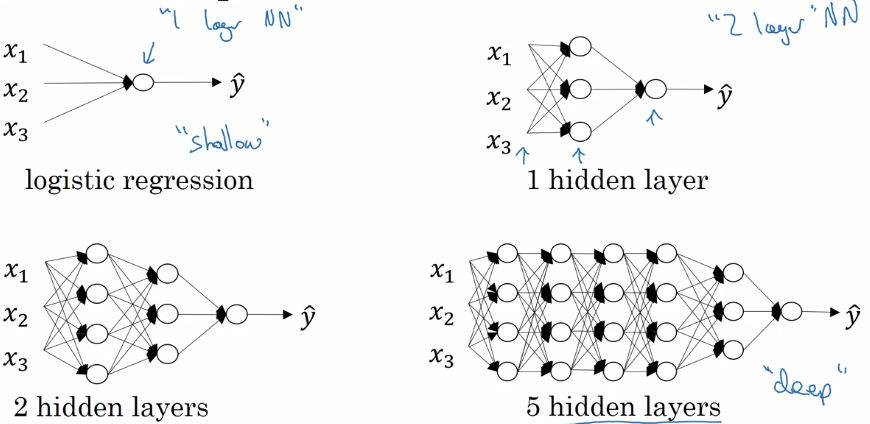notation: layer 0 = input layer `L` = number of layers `n^[l]` = size of layer l `a^[l]` = activation of layer l = `g[l]( z[l] )` → a[L] = yhat, a = x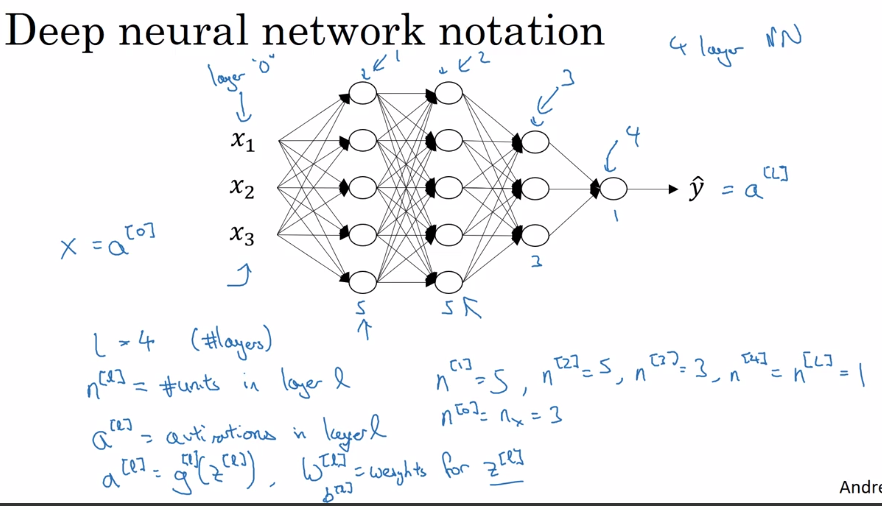## Forward Propagation in a Deep Network⇒ general rule: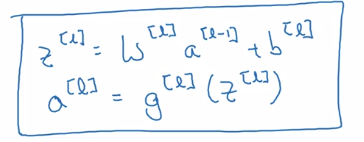vectorization over all training examples: Z = [z(1),...,z(m)] one column per example ⇒

```A = X
for l = 1..L:
Z[l] = W[l]A[l-1] + b[l]
A[l] = g[l]( Z[l] )
Yhat = A[L]
```

## Getting your matrix dimensions right

Debug: walk through matrix dimensions of NN, `W[l]`.

Single training example dimension:
`a[l-1].shape = (n[l-1], 1)`
`z[l].shape = (n[l], 1)`
`z[l] = W[l] * a[l-1] + b[l], shape = (n[l],1)`
W[l].shape = (n[l], n[l-1]), b[l].shape = (n[l],1)Vectorized (m examples) dimension:
Z = [z(1),...,z(m)] stacking columns.
`Z[l].shape = (n[l], m)`
Z[l] = W[l] * A[l-1] + b[l]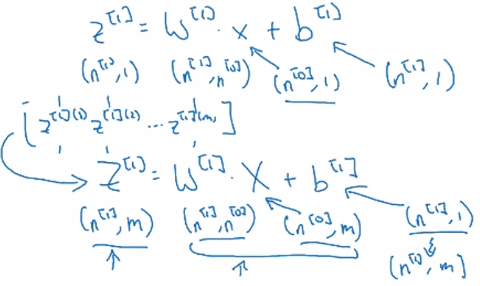Z[l].shape = A[l].shape = (n[l], m)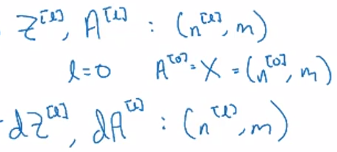## Why deep representations?

intuition: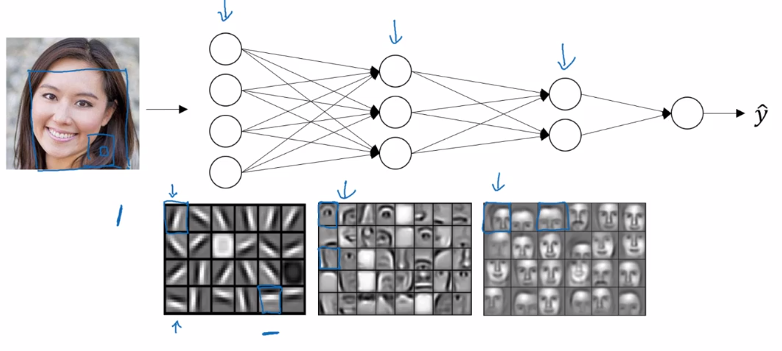as layers grow: simple to complex representation / low to high level of abstraction.

Circuit theory: small deep NN is better than big shallow NN.Example: representation of a XOR.join(x1..xn) function.

• Using deep NN ⇒ build an XOR binary tree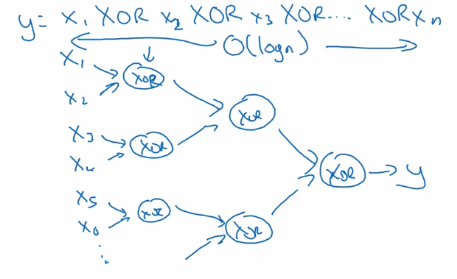• Using shallow NN: one single layer → enumerate all 2^n configurations of inputs.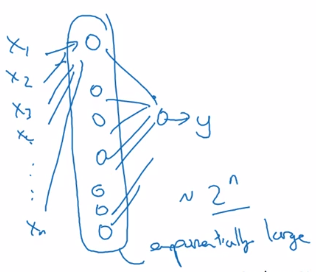## Building blocks of deep neural networks

Fwdprop and backprop, for layer l.

• Fwdprop: from a[l-1] to a[l]

note: cache z[l] for backprop.

• Backprop: from da[l] to da[l-1], dw[l] and db[l]Once the fwd and back functions are implemented, put layers together:## Forward and Backward Propagation

Fwd prop
input = a[l-1], output = a[l], cache = z[l]

```Z[l] = W[l] * A[l-1] + b[l]
Z[l] = g[l]( Z[l] )
```

Back prop input = da[l], output = da[l-1], dW, db[l]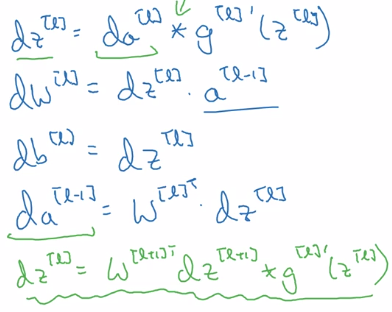note:

• remember `da = dL/da`, so here `da`~='1/da' mathematically.
• derivate of matrix multiplication = transposed matrix derivative: (A*B)' = B^T' * A^T'
• initial paule of backprop: da[L] = dL/dyhat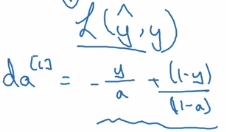Vectorized version:## Parameters vs Hyperparameters

• parameters: W[l] and b[l] → trained from data
• hyperparams:
• alpha (learning_rate), number of iterations, L, n[l] size of each layer, g[l] at each layer...
• momentum, minibatch, regularization...

→ finally decides what params will be.

empirical: try out different hyperparams.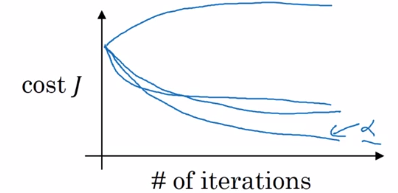## What does this have to do with the brain?

logistic regression unit ~~~> neuron in brain

## assignment: implementing a L-layer NN

• params initialization:

note: different signature for `np.random.randn` and `np.zeros`:

```W = np.random.randn(d0, d1) * 0.01
b = np.zeros((d0, d1)) # Needs putting dims in a tuple!
```
• function activation:

`np.maximum` is element-wise comparison, whereas `np.max` will apply on certain axis. so `ReLU(x) = np.maximum(0, x)`

• Fwd prop:• cost:• backprop formula: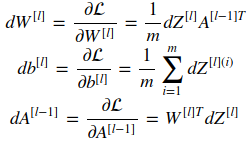• initial paulse of backprop dA[L]: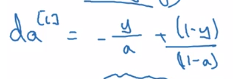`dAL = - (np.divide(Y, AL) - np.divide(1 - Y, 1 - AL))`

#### Part 4 of series «Andrew Ng Deep Learning MOOC»：

comments powered by Disqus